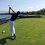# $\huge{"}$unfactorable$\huge{"}$Alright, I guess you could call it a "pet peev" but when ever I hear the word unfactorable, it just hits a nerve, because in reality, all equations are factorable...technically.

The most stereotypically "unfactorable"... Or should I say "not pretty", equation is $x^2-x-1$. WELL GUESS WHAT you can factor it as $(x-\frac{1}{2}-\frac{\sqrt5}{2})(x-\frac{1}{2}+\frac{\sqrt5}{2})$.

Yes, it sure isn't pretty, but it works. You can use the quadratic equation and match the first coefficients. This will work sometimes, but sometimes, you must use a little more unconventional ways.

Apparently, every teacher has this deadly disease called "unfactorablus polynomium" (lol) because not one teacher at our school taught us this.

I mean, when I say that EVERY polynomial is factorable, I mean EVERY SINGLE ONE.

💭💭💭. "what about $x-5$?"

Not factorable???? Nope, too bad, sorry math, I win, $\textit{it is}$. Just use difference of cubes.

$x-5$

$(^3\sqrt{x}- ^3\sqrt5)(^3\sqrt{x^2}+^3\sqrt{5x}+^3\sqrt{5^2})$

There, it's done being factored.... WRONG... I see a sum of squares and a difference of squares.

$(^6\sqrt{x}-^6\sqrt5)(^6\sqrt{x}+^6\sqrt5)(^6\sqrt{x^2}+i\sqrt{^3\sqrt{5x}+^3\sqrt{5^2}})(^6\sqrt{x^2}-i\sqrt{^3\sqrt{5x}+^3\sqrt{5^2}})$

But you get the point. I could go on forever using these BRILLIANT factoring Lemmas... But turns out, that only one of them actually has a 0.. So there will only be one intercept because the original equation only has one root. In fact, none of the others even have imaginary roots. Thus the original equation is not changed.

So I ask of you all to please spread the knowledge of the Unfactorablus Polynomium disease. And remember:

"I don't care what it is, everything is factorable......unless it's a potato" -Trevor Arashiro 2014.

Don't forget to hashtag your posts

# . #everythingisfactorable #factoringforthewin #fundamentaltheoremofalgebraiswrong #cuztrevorsaidsoNote by Trevor Arashiro
6 years, 9 months ago

This discussion board is a place to discuss our Daily Challenges and the math and science related to those challenges. Explanations are more than just a solution — they should explain the steps and thinking strategies that you used to obtain the solution. Comments should further the discussion of math and science.

When posting on Brilliant:

• Use the emojis to react to an explanation, whether you're congratulating a job well done , or just really confused .
• Ask specific questions about the challenge or the steps in somebody's explanation. Well-posed questions can add a lot to the discussion, but posting "I don't understand!" doesn't help anyone.
• Try to contribute something new to the discussion, whether it is an extension, generalization or other idea related to the challenge.

MarkdownAppears as
*italics* or _italics_ italics
**bold** or __bold__ bold
- bulleted- list
• bulleted
• list
1. numbered2. list
1. numbered
2. list
Note: you must add a full line of space before and after lists for them to show up correctly
paragraph 1paragraph 2

paragraph 1

paragraph 2

[example link](https://brilliant.org)example link
> This is a quote
This is a quote
    # I indented these lines
# 4 spaces, and now they show
# up as a code block.

print "hello world"
# I indented these lines
# 4 spaces, and now they show
# up as a code block.

print "hello world"
MathAppears as
Remember to wrap math in $$ ... $$ or $ ... $ to ensure proper formatting.
2 \times 3 $2 \times 3$
2^{34} $2^{34}$
a_{i-1} $a_{i-1}$
\frac{2}{3} $\frac{2}{3}$
\sqrt{2} $\sqrt{2}$
\sum_{i=1}^3 $\sum_{i=1}^3$
\sin \theta $\sin \theta$
\boxed{123} $\boxed{123}$

Sort by:

Maybe teachers should mention the Fundamental Theorem of Algebra, for the sake of completeness.

- 6 years, 9 months ago

So ... I was watching this new show called Scorpion, about a bunch of high-IQ misfits problem solving think-tank

In a flashback, one of them had a teacher who wrote several numbers on the board, including 703.
Teacher: "Which of these numbers is divisible by 4?"
Student: "All of them."
Teacher: "Wrong! 703 is not ..."
Student: "703 divided by 4 is 175.75. You did not define your terms properly."

Staff - 6 years, 9 months ago

Haha. That is one cool guy.

- 6 years, 9 months ago

Excellent to read and easy to understand this mathmatically operation as your post which should be some improvement and suggestion from experts. I really happier to read it and get valuable knowledge and now it's too easier to get Edugeeksclub dissertation writing help from expert writers.

- 2 years, 2 months ago